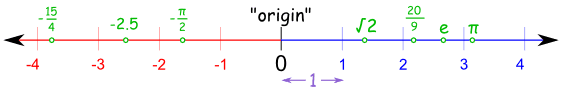# GMAT Quant: Arithmetic – Real NumbersMost-tested and most favorite topic on the GMAT Quantitative Section is “Number Properties.” Sums on Real Number falls in this huge category of Number properties.

## What are Real Numbers?

Nearly all numbers that you can think of is a real number. Real numbers is a superset of whole numbers (0, 1, 2, 3…..), natural numbers (1, 2, 3…), irrational (π/2, √3, etc.) and rational numbers (2/3, 1.6666, etc.).

Real numbers can be either positive, negative or zero. Whereas, infinity and imaginary numbers like √−1, square root of minus 1, are not a part of real numbers.

## Properties of Real Numbers

Any real number can be shown on the real number line.

A real number line is line on which any rational, irrational, integer etc. can be shown. An origin is selected as the point of reference and values on the right of origin are said to be positive whereas, to the left is negative values.This picture is of a real number line showing different values.

Real numbers are commutative, distributive and associative in nature.

Commutative property

X + Y = Y + X

Example;

7 + 8 = 8 + 7

Distributive property

X * (Y + Z) = XY + YZ

Example;

9 * (5 + 10) = 9 * 5 + 9 * 10

Associative property

(X + Y) + Z = X + (Y + Z)

Example;

(29 + 10 ) + 12 = 29 + (10 + 12)

Real numbers have the closure property as well.

Means, if X is a real number and,

If Y is a real number,

Then, X + Y and X * Y will also be a real number.